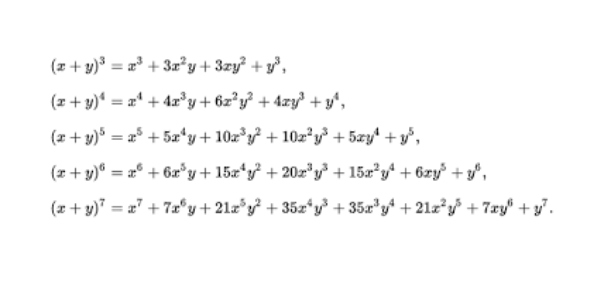# Newton Discovered - Binomial Theorem Quiz

12 Questions | Attempts: 143
ShareSettingsNewton DiscoveredThe Binomial Theorem Quiz

• 1.
Pascal's Triangle was first discovered by Blaise Pascal.
• A.

Not True

• B.

Not False

• 2.
Who was born first, Isaac Newton or Blaise Pascal?
• A.

Blaise Pascal

• B.

Isaac Newton

• C.

They were born at the same time

• 3.
The coefficients that appear in the binomial expansion are called ____ ____.
• 4.
Newton's contributions to mathematics include:
• A.

Differential Calculus

• B.

Integral Calculus

• C.

Accumulative Calculus

• D.

Binomial Theorem

• E.

Gravitational Theory

• F.

Pascal's Triangle

• G.

Astronomy

• 5.
The expansion of (x+y)n has ____ number of terms.
• A.

(n+2)

• B.

(n+1)

• C.

N

• 6.
The fifth term of the expansion of (x+2)5 is ____.
• A.

16

• B.

32x

• C.

16x

• D.

32

• 7.
Find the coefficient of the term in x5 in the expansion of (x2-1/2x3)5.
• A.

-2.5

• B.

-2

• C.

1/8

• D.

-0.125

• E.

4.5

• 8.
In the expansion (3x-0.2x-1)8, find the term independent of x.
• A.

-0.6

• B.

8.426

• C.

-0.256

• D.

9.072

• E.

1134/125

• 9.
(r+1)th term = nCr an-r br
• A.

Not True

• B.

Not False

• 10.
The number of permutations is not r! times as much as the number of combinations in nCr.
• A.

Not True

• B.

Not False

• 11.
Newton discovered binomial theorem in the year ____.
• 12.
This triangle is known as:
• A.

China's Triangle

• B.

Pascal's Triangle

• C.

Chinangle

• D.

Yang Hui's Triangle

• E.

Triangle of Confucius

## Related TopicsBack to top
×

Wait!
Here's an interesting quiz for you.